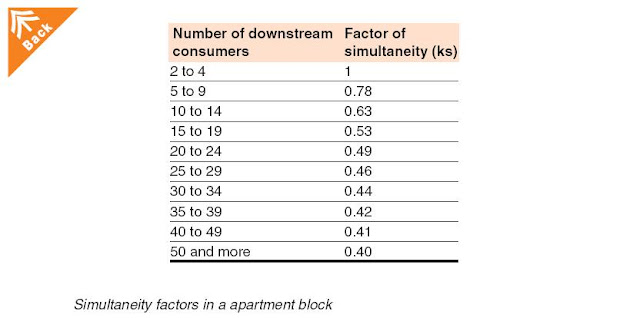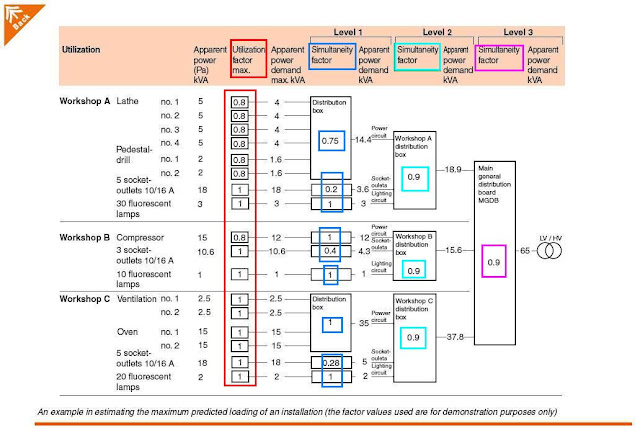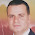Electrical Load Estimation – Part One

At the beginning of the project,in the draft design (early design) stage,  the electrical design professional should do the following:

• Make Analysis of load characteristics,
• Review The available voltage system types/classes and levels.
• Review the utility’s rate structure,
• Make roughly a key single-line diagram and a set of subsidiary single-line diagrams. The key single-line diagram should show the sources of power e.g. generators, utility intakes, the main switchboard and the interconnections to the subsidiary or secondary switchboards.
• Develop Demand factor relationship between connected loads and the actual demand imposed on the system,

Importance of Electrical Load Estimation ( preliminary load calculations)

Electrical Load Estimation is very important in the draft design (early design) stage because it help to:

• Plan the connection to upstream network and MV circuit configurations.
• Plan the transformers substation(s) (if any) and the main switchgear room.
• Apply to Power Company for supply.
• Calculate initial budget for the electrical works.

Definition of Important terms in Load Estimation:

there are many Important terms which must be understood before performing the load estimation, these terms are:

It is the Sum of all the loads connected to the electrical system, usually expressed in watts.

It is The electric load at the receiving terminals averaged over a specified demand interval of time, usually 15 min., 30 min., or 1 hour based upon the particular utility’s demand interval. Demand may be expressed in amperes, kilo-amperes, kilo-watts, kilo-vars, or kilo-volt-amperes.

3- Demand Interval

It is The period over which the load is averaged, usually 15 min., 30 min., or 1 hour.

4- Maximum demand

It is The greatest of all demands that have occurred during a specified period of time such as 5 minutes, 15 minutes, 30 minutes or one hour. For utility billing purposes the period of time is generally one month.

5- Demand factor (in IEC , Factor of maximum utilization ku)

In normal operating conditions the power consumption of a load is sometimes less than that indicated as its nominal power rating.

The demand factor is the ratio of the maximum demand on a system to the total connected load of the system.

Demand factor = Maximum demand load / Total load connected

Notes:

• This factor must be applied to each individual load, with particular attention to electric motors, which are very rarely operated at full load.
• Demand factors for buildings typically range between 50 and 80 percent of the connected load. For most building types, the demand factor at the service where the maximum diversity is experienced is usually 60 to 75 percent of the connected load. Specific portions of the system may have much higher demand factors, even approaching 100 percent.

6- Coincidence factor (in IEC, Factor of simultaneity ks)

It is a matter of common experience that the simultaneous operation of all installed loads of a given installation never occurs in practice, i.e. there is always some degree of diversity and this fact is taken into account for estimating purposes by the use of a simultaneity factor (ks).

The coincidence factor is the ratio of the maximum demand of a system, or part under consideration, to the sum of the individual maximum demands of the subdivisions.

Coincidence factor = Maximum system demand / Sum of individual maximum demands

Notes:

• The factor ks is applied to each group of loads (e.g. being supplied from a distribution or sub-distribution board).

Example#1 (see Fig.1 ):

5 storeys apartment building with 25 consumers, each having 6 kVA of installed load.

calculate the following:
• The total installed load
• The apparent-power supply
• the main service size
• the third level service sizeFig (1)

the solution:

• The total installed load for the building is: 36 + 24 + 30 + 36 + 24 = 150 kVAtable#1

From table#1 in above, it is possible to determine the magnitude of currents in different sections of the common main feeder supplying all floors. For vertical rising mains fed at ground level, the cross-sectional area of the conductors can evidently be progressively reduced from the lower floors towards the upper floors. T
hese changes of conductor size are conventionally spaced by at least 3-floor intervals.

• so, The apparent-power supply required for the building is: 150 x 0.46 = 69 kVA

• the current entering the rising main at ground level( main service size) is:

(150 x 0.46 x 1000) / (400 x √3) = 100 A

• The current entering the third floor (the third level service size) is:

(36 + 24) x 0.63 x 1000 / (400 x √3) = 55 A

7- Diversity factor

The diversity factor is the reciprocal of the coincidence factor.
Diversity factor = Sum of individual maximum demands / Maximum system demand

Notes:

• The Diversity Factor is applied to each group of loads (e.g. being supplied from a distribution or sub-distribution board).

Example#2:
Consider that a feeder supplies five users with the following load conditions:
• On Monday, user one reaches a maximum demand of 100 amps;
• on Tuesday, two reaches 95 amps;
• on Wednesday, three reaches 85 amps;
• on Thursday, four reaches 75 amps;
• on Friday, five reaches 65 amps.
• The feeder’s maximum demand is 250 amps.

Calculate the Diversity Factor for this feeder?

the solution:

The diversity factor can be determined as follows:

Diversity factor = Sum of total demands ÷ Maximum demand on feeder = 420 ÷ 250 = 1.68

Example#3:
Calculate the size of a main feeder from substation switchgear that is supplying five feeders with connected loads of 400, 350, 300, 250 and 200 kilovolt-amperes (kVA) with demand factors of 95, 90, 85, 80 and 75 percent respectively. Use a diversity factor of 1.5.

the Solution
1- Calculate demand for each feeder:

• 400 kVA × 95% = 380 kVA
• 350 kVA × 90% = 315 kVA
• 300 kVA × 85% = 255 kVA
• 250 kVA × 80% = 200 kVA
• 200 kVA × 75% = 150 kVA

2- sum all of the individual demands = 1,300 kVA

3- If the feeder were sized at unity diversity, then 1,300 kVA ÷ 1.00 = 1,300 kVA

However, using the diversity factor of 1.5, the kVA = 1,300 kVA ÷ 1.5 = 866 kVA for the feeder.

Difference between demand and diversity factor:

most of the electrical engineers confuse between the demand and diversity factors, to solve this confusion, don't forget that:

• The Demand factor must be applied to each individual load, with particular attention to electric motors, which are very rarely operated at full load.
• The Diversity Factor is applied to each group of loads (e.g. being supplied from a distribution or sub-distribution board).

Example #4:
An industrial building consists of (3) nos. workshops A, B & C, each workshop will include the following loads:
Workshop A:

• 4 nos. lathe with 5 KVA each,
• 2 nos. pedestal drill with 2 KVA each,
• 5 nos. sockets outlets 10/16 A on one circuit with 18 KVA total,
• 30 nos. fluorescent lamps on one circuit with 3 KVA total.
Workshop B:

• One nos. Compressor with 15 KVA,
• 3 nos. sockets outlets 10/16 A on one circuit with 10.6 KVA total,
• 30 nos. fluorescent lamps on one circuit with 1 KVA total.

Workshop C:
• 2 nos. ventilation fans with 2.5 KVA each,
• 2 nos. Oven with 15 KVA each,
• 5 nos. sockets outlets 10/16 A on one circuit with 18 KVA total,
• 20 nos. fluorescent lamps on one circuit with 2 KVA total.

• Draw a key single line diagram for this building?
• determine both the demand (utilization) factor and simultaneity factor with the help of tables # 2 & 3 in below?
• Calculate the demand load for each level in the key single line diagram?table#2table#3

The solution:

follow the solution steps in below and in fig.2.fig.2

Step#1: List all the loads in each workshop and write the apparent power of each load in KVA beside it.

Step#2: write the utilization factor for each load , IEC gives Ku estimation values for these loads as follows:

• For motor Ku = 0.8
• For socket outlets Ku = 1 (depend on the type of appliances being supplied from the sockets concerned)
• For light circuits Ku= 1

Step#3: calculate the Max. demand apparent power in KVA for each load = apparent power X Ku for each load.

Step# 4: group same type of loads on one distribution panel/box and this will be the first Level of distribution (LEVEL 1).

Step# 5: in level 1 and from table #2, write the simultaneity factor for each distribution panel/box and from table # 3 write the simultaneity factor for each for each separate load.

Step# 6: calculate the Max. demand apparent power in KVA for each distribution panel/box = sum of all branch loads’ Max. demand apparent power in KVA X simultaneity factor for each distribution panel/box.

Step# 7: group the distribution panel/box in each workshop in one main distribution panel/box. So, we will have (3) main distribution panel/box for the (3) workshops and this will be the second level of distribution (LEVEL 2).

Step# 8: in level 2 and from table #2, write the simultaneity factor for each main distribution panel/box.

Step# 9: calculate the Max. Demand apparent power in KVA for each main distribution panel/box = sum of all branch distribution boxes’ Max. Demand apparent power in KVA X simultaneity factor for each main distribution panel/box.

Step# 10: group the (3) main distribution panel/box in one main general distribution board MGDB and this will be the third level of distribution (LEVEL 3).

Step# 11: in level 3 and from table #2, write the main general distribution board MGDB.

Step# 12: calculate the Max. Demand apparent power in KVA for main general distribution board MGDB = sum of the (3) workshop main distribution boxes’ Max. Demand apparent power in KVA X simultaneity factor for main general distribution board MGDB.

The load factor is the ratio of the average load over a designated period of time, usually 1 year, to the maximum load occurring in that period.

you can download tables for different factors listed above by clicking the following links:

Methods of Electrical load estimation

There are (5) methods for Electrical Load Estimation, which are:

A- Preliminary load calculation which divide to:

1. Space by space (functional area method),
2. Building Area method.
3. Area method.

B- NEC load calculations.

C- Final load calculations.

In the next topic, I will explain these methods for Electrical load estimation. so, please keep following.

1.This is a very good electrical engineering course.

2.i can not understand the level 1 ,
as why you take .75 from table 2 " why you assumed that it is the second most powerful motor ??
and why you take for the socket once by 0.2 and once by 0.4 and once by 0.28???
and can you upload this table in word please or a HD pic ??

3.to medo88:
1- please note that in below of picture for the example, it is written that the values in this example are for demonstration only.but when you have a real load estimation, you must use the values included in the tables.

2- Ks= 0.75, it is taken from table 2 as the number of circuits = (4) lathe + (2) pedestal drill = (6)and from table 2 the Ks will be 0.7 but you can use higher value especially in industrial installations.

3- Ks for sockets is determined from table 3 to be from 0.1 to 0.2 but you can put higher values as in note#1 in the same table.

3- please note that these calculations is estimation only, you can use higher values (not lower) than that written in the tables as per your judgement.

5- feel free to ask me again, if my answers are not clear for you.

4.thank you eng ali for your answer , as i was waiting for it .

5.Hello there Mr. Ali,

Are the values of Utilization factor (Ku) & Simultaneity factor (Ks) for each & every types of Load. What is the IEC series number for this?

1.yes, there are values of Utilization factor (Ku) for each type of Load and Simultaneity factor (Ks)for each group of identical loads.
Factor of simultaneity for distribution boards (IEC 61439)
Diversity Factor for according to circuit function (IEC 60439)

6.7.